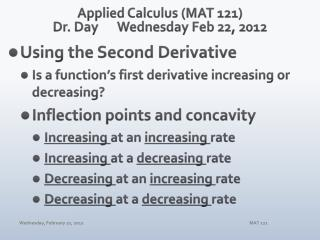DownloadDownload PresentationApplied Calculus (MAT 121) Dr. Day Wednes day Feb 22, 2012

# Applied Calculus (MAT 121) Dr. Day Wednes day Feb 22, 2012

Download Presentation## Applied Calculus (MAT 121) Dr. Day Wednes day Feb 22, 2012

- - - - - - - - - - - - - - - - - - - - - - - - - - - E N D - - - - - - - - - - - - - - - - - - - - - - - - - - -
##### Presentation Transcript

1. Applied Calculus (MAT 121)Dr. Day Wednesday Feb 22, 2012 • Using the Second Derivative • Is a function’s first derivative increasing or decreasing? • Inflection points and concavity • Increasing at an increasing rate • Increasing at a decreasing rate • Decreasing at an increasing rate • Decreasing at a decreasing rate MAT 121

2. What does the second derivative tell us? • Not only can a function increase or decrease, it can do so in various ways. The second derivative helps us identify patterns in how a function increases or decreases. These patterns help us describe the concavity of a function. • The key points where concavity changes are called points of inflection. The second derivative helps us identify these key points. • Specific contextual situations are more thoroughly analyzed using the second derivative. Connections among position, velocity, and acceleration can be made using first and second derivatives. MAT 121

3. MAT 121

4. The Second Derivative Reveals a Function’s Concavity Example: Determine the intervals on which the function f(x) = x3 – 3x2 – 24x + 32 is concave up and on which it is concave down. Identify all points of inflection. MAT 121

5. The Second Derivative Reveals a Function’s Concavity Example: Determine the intervals on which the function f(x) = x+ 1/xis concave up and on which it is concave down. Identify all points of inflection. MAT 121

6. Assignments WebAssign 4.1 due tonight. 4.2 due Thursday 2/23. Reminder: Check results for Test #2 MAT 121